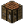# VariablesTutorial: Variables This is a tutorial page for Variables and their function. Objective To gain an understanding of how Variables work. Prerequisites A basic understanding of programming in ComputerCraft.

## What are Variables?

Variables are one of the fundamental building blocks of all coding languages. Variables are just as they sound, they can store a changeable value.

## What do variables look like?

Variables will usually be defined near the start of a script or function (Functions will be taught in a later tutorial), as this will make them easy to edit later if required. Variables can be defined in a number of different ways, reliant on the type of data that is being stored.

String values

String variables can store any text or "string" that you will use in your script. Strings can be defined as follows

```local Text = 'This is a string!'
local MoreText = "This is also a string!"
```

String values have the unique ability to be concatenated (One added to the end of the other). To do this, simply enter a ".." between the two strings or variables.

```local Start = "This is"
local Middle = " all part of "
local End = "one sentence!\n"

Start = Start..Middle

write(Start..End)
```

This will write "This is all part of one sentence!"

Number values

Number variables can store any number used in the script, as well as perform calculations with them. Numbers can be defined as follows

```local Number = 1
local AnotherNumber = 2
```

Lua supports the following mathematical functions:

• Addition (+) 1+1 = 2
• Subtraction (-) 2-1 = 1
• Multiplication (*) 2*2 = 4
• Division (/) 2/2 = 1
• Modulus (%) 5%2 = 1
• Power (^) 2^3 = 8

Boolean values

Boolean variables are simply a "True or False?" variable. They can be either true or false, but nothing else. Boolean values are usually used for conditional statements, which will be covered in a later tutorial. Boolean variables can be defined as follows

```local Bool = true
local Bool2 = false
```

Note that "true" and "false" are case sensitive.

Nil values

Nil values are values that are currently storing nothing. Usually you will not explicitly set a variable to nil, as it acts similar to a "false" boolean. All variables are nil by default.

```local Variable = nil
```

Note that "nil" is case sensitive

Table values

Table variables are variables that can store any other variables, including more tables. They will be described in more depth in a later tutorial. Tables can be defined as follows

```local EmptyTable = {}
local Table = {1,2,3,4}
local AnotherTable = {"This table", "Contains", 3, {"Types of variables!"} }
```

Function values

Although not often considered variables, Lua functions can act the same way as other more standard variables. Functions will be described in more detail in a later tutorial. functions can be defined as follows

``` local DoStuff = function() end
local function AnotherFunction() end

local DoStuff2 = function()
write("Function!\n")
end
local function AnotherFunction2()
write("Another function!\n")
end
```

Function and table variables are usually defined over multiple lines due to the length of them. In Lua, a new line does not mark any functionality and is used purely for readability.

Unlike many other languages, Lua allows for variable's data types to be changed as you go, easily overwriting, for example, a string with a number.

``` local Variable = "String!" --The variable is a string
Variable = 1 --The variable is now a number
```

## What is "local" and why is it useful?

You may have noticed the use of "local" in the above declarations. "local" simply states that the variable being defined should only be used in this script or function. You only need to write local for the first time you set a variable, then it will assume every other time you use the variable afterwards it is the local variable. If you use or set a variable without using local, it will try and use or set a global variable which can be used and modified by other scripts.

Although it likely won't be an issue when you are using ComputerCraft, it is advisable to use local variables and functions when possible, so they will not be unintentionally edited by other functions or scripts.

## Multiple assignments

Variables don't necessarily need to be defined one at a time, it is possible to assign multiple values in one operation through the use of commas.

``` local Var1, Var2 = "One", "Two"
write( Var1..Var2.."\n" )
```

This script will successfully assign the values "One" and "Two", then write "OneTwo"

## What have we learned?

• Variables can store values
• Variables store different types of values
• Variables can be defined in different ways
• An overview of what different variable types do
• Use "local" to use variables locally
• Multiple values may be assigned at once